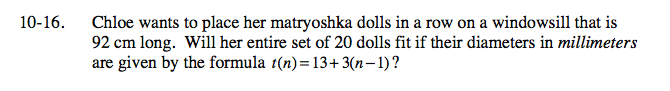Home > CCA2 > Chapter 10 > Lesson 10.1.1 > Problem10-16

10-16.

Chloe wants to place her matryoshka dolls in a row on a windowsill that is 92 cm long. Will her entire set of 20 dolls fit if their diameters in millimeters are given by the formula t(n) = 13 + 3(n − 1)? Homework Help ✎Graph the series from t(1) to t(20).

Rearrange the graph into a single rectangle and find the area.

Is the total less than 92 cm?

Pay careful addition to units. How many millimeters are in a centimeter?